Courses

# SSC CPO Mock Test (GI & Reas) 9

## 50 Questions MCQ Test SSC CPO & Constable - Mock Tests & Previous Year Papers | SSC CPO Mock Test (GI & Reas) 9

Description
This mock test of SSC CPO Mock Test (GI & Reas) 9 for SSC helps you for every SSC entrance exam. This contains 50 Multiple Choice Questions for SSC SSC CPO Mock Test (GI & Reas) 9 (mcq) to study with solutions a complete question bank. The solved questions answers in this SSC CPO Mock Test (GI & Reas) 9 quiz give you a good mix of easy questions and tough questions. SSC students definitely take this SSC CPO Mock Test (GI & Reas) 9 exercise for a better result in the exam. You can find other SSC CPO Mock Test (GI & Reas) 9 extra questions, long questions & short questions for SSC on EduRev as well by searching above.
QUESTION: 1

Solution:
QUESTION: 2

Solution:
QUESTION: 3

### Find out which word or name that will be in the middle after the words or names are arranged in the alphabetical order.

Solution:
QUESTION: 4
Choose the number series which is different from others.
Solution: All other pairs except ( 37, 14, 19, 7) consists of prime numbers only,
while (37, 14, 19, 7) consists of one composite number i.e., 14
QUESTION: 5
Choose the word which is least like the other words in the group .
Solution:
QUESTION: 6
Choose the numeral pair which is different from others .
Solution:
QUESTION: 7
Complete the analogous pair.
King : Royal :: ? : Religious
Solution:
QUESTION: 8
Complete the analogous pair.
Mature : Regressed : : Varied : ?
Solution:
QUESTION: 9
Complete the analogous pair.
Dilatory : Expeditious : : Direct : ?
Solution:
QUESTION: 10
Complete the analogous pair.
Grapes : Wine : : Wheat : ?
Solution:
QUESTION: 11
A is 40 m South-west of B. C is 40 m South-east of B. Then, C is in which direction of A ?
Solution:
QUESTION: 12

If the order of first half of the letters in the English alphabet is reversed, which letter will be eighth to the left of fifteenth letter from the left?

Solution:
QUESTION: 13
How many rectangles are there in the given figure.
Solution:
QUESTION: 14

Find the missing character.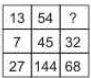Solution:

In the first column, 13 + 7 x 2 = 27.
In the second column, 54 + 45 x 2 = 144. Let the missing number in the third column be x.
Then, x + 32 x 2 = 68 o r x = 68 − 64 = 4.

QUESTION: 15
A is 40 m south-west of B. C is 40 m south-east of B. then C is in which direction of A?
Solution:
C is to the East of A
QUESTION: 16
Find the missing character.
Solution: The numbers in the first row form a series 12, 22, 32.
So, missing number in the first row =42=16.
The numbers in the second row form the series, 1, 2, 3, 4.
The numbers in the third row form the series 2, 4, 6.
So, missing number in the third row =6+2=8.
QUESTION: 17
Group the following figures into three classes on the basis of identical properties.
Solution:
QUESTION: 18
Find the missing character.
Solution:
QUESTION: 19

Choose the odd one out.

Solution:
QUESTION: 20

Choose the odd one out.

Solution:
QUESTION: 21

How many such pairs of letters are there in the word DELIBERATE which have as many letters between them in the word as in the alphabet?

Solution:

1 - RAT - RST
2 - DE - DE
3 - ELIB - EDCB
4 - ETAREBIL - EFGHIJKL

QUESTION: 22

In a certain code BROWN is written as CSPXO, How is PART written in that code?

Solution:

The pattern is writing the next letter
i.e. PART → QBSU

QUESTION: 23

In a certain code ROPE is written as \$3%6 and RITE is written as \$4#6. How is PORT written in that code?

Solution:
QUESTION: 24
In a certain code language CAT is coded as 24, what will be the code number for BAT in the same code language ?
Solution:
QUESTION: 25

If '*' means '×', \$ means '+', '#' means '÷' and '©' means '-' then the what is the value of 360 # 24 \$ 56 * 5 © 48 ?

Solution:

360 ÷ 24 + 56 × 5 - 48
⇒ 15 + 280 - 48 = 247

QUESTION: 26
Complete the analogous pair.
Given set : (81, 77, 69)
Solution:
QUESTION: 27
Find the odd number from the given alternatives.
Solution:
QUESTION: 28
Choose the odd one out.
Solution:
QUESTION: 29

Choose the odd one out.

Solution:

1 : 4
1 × 2 + 2 = 4
8 : 18
8 × 2 + 2 = 18
22 : 46
22 × 2 + 2 = 46
50 : 102
50 × 2 + 2 = 102
10 : 24
10 × 2 + 4 = 24

QUESTION: 30
Use the following series of elements (alpha number symbol) to answer these questions. Every two digit number (given in brackets) is to be treated as single number.
2 * 8 5 6 B 9 \$ Q 3 E 1 7 R D 4 (13) U∙ K (18) A (14) P
Four of the following five groups of elements are alike in a certain way and so form a group. Which is the one that does not belong to that group?
Solution:
QUESTION: 31
Which term will replace the question mark in the series :
ABD, DGK, HMS, MTB, SBL, ?
Solution:
QUESTION: 32
Find out from amongst the four alternatives as to how the pattern would appear when the transparent sheet is folded at the dotted line.
Solution:
QUESTION: 33
Choose the correct alternative that will continue the same pattern and fill in the blank.
1, 2, 3, 5, 8, (....)
Solution:
QUESTION: 34
Find the wrong number in the series.
3, 4, 13, 38, 115, 166
Solution:
QUESTION: 35
Choose the correct alternative that will continue the same pattern and fill in the blank.
2, 15, 41, 80, (.....)
Solution:
QUESTION: 36
Which number would replace question mark in the series 7, 12, 19, ?, 39.
Solution:
QUESTION: 37
Below is given statement followed by two assumptions numbered I and II. You have to consider the statement and the following assumptions and decide which of the assumptions is implicit in the statement :
Statement : "We should revise the wage structure in order to retains our good employees."-Comment of a member of the Management Committee of a company
Assumptions:
I. Wage structure of other companies involved in parallel activities are better.
II. Wage structure is one of the most important factors for the employees of the company.
Solution:
QUESTION: 38
Below is given statement followed by three conclusions numbered I, II and III. You have to consider the statement and the following conclusions and decide which of the conclusions is follows in the statement :
Statements :a. Some picture are frames.
b. Some frames are idols.
c. All idols are curtains.
Conclusions:I. Some curtains are pictures.
II. Some curtains are frames.
III. Some idols are frames.
Solution:
QUESTION: 39

If 'see' means 'hear', 'hear' means 'feel', 'feel' means 'smell' and 'smell' means 'see' then what are ears used for?

Solution:
QUESTION: 40
In each of the following questions, four groups of three words each are given. Only one group in each does not share the underlying principle that combines the words. Find that group:
Solution:
QUESTION: 41

Ashish leaves his house at 20 minutes to seven in the morning, reaches Kunal`s house in 25 minutes, they finish their breakfast in another 15 minute and leave for their office which takes another 35 minutes, At what time do they leave Kunal`s houses to reach their office ?

Solution:

Ashish leaves his house at 6.40 a.m. He reaches Kunal`s in 25 minutes i.e. 7.05 a.m. Both leave for office 15 minutes after 7.05 a.m. i.e. at 7.20 a.m.

QUESTION: 42
How many meaningful word is possible with the second, the fifth, the tenth and the twelfth letters of the word 'METROPOLITAN'? If no such word can be made, give X as the answer and if more than one such word can be made, given M as the answer.
Solution: The second, fifth, tenth and twelfth letters of the word METROPOLITAN are E, O, T and N respectively. The words formed are NOTE and TONE.
QUESTION: 43

In the following questions, select a figure from amongst the four alternatives, which when placed in the blank space of fig. (X) would complete the pattern.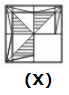Solution:
QUESTION: 44

In each of the following question, three classes of persons, objects or streams are given. Each class can be represented by one of the seven Venn diagrams given below. Indicate which figure best represents the relationship among three classes?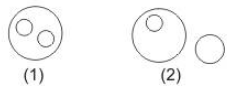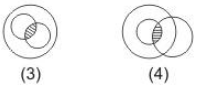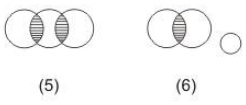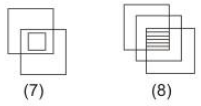Q. Which of the following Venn diagrams best illustrates the three classes :
Antibiotic
Antiseptic
Antibody

Solution:

(6) Antibiotic and Antiseptic are inter-related medicines.
Antibody is different as internal formations in human body.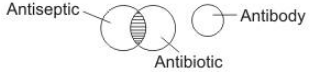QUESTION: 45

In each of the following question, three classes of persons, objects or streams are given. Each class can be represented by one of the seven Venn diagrams given below. Indicate which figure best represents the relationship among three classes?Q.  Which of the following Venn diagrams best illustrates the three classes :
Fear
Anxiety
Nervousness

Solution:

(8) All three are inter-related as an uneasy mental state.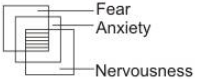QUESTION: 46

In each of the following questions, which one of the for interchanges in signs and numbers would make the given equation correct?

29. 6 × 4 + 2 = 16

Solution:

On interchanging + and × and 4 and 6, we get the equation as
4 + 6 × 2 = 16
or 4 + 12 = 16
or 16 = 16, which is true

QUESTION: 47

In each of the following questions, which one of the for interchanges in signs and numbers would make the given equation correct?

30. (3 ÷ 4) + 2 = 2

Solution:

On interchanging + and ÷ and 2 and 3, we get the equation as
(2 + 4) ÷ 3 = 2
or 6 ÷ 3 = 2
or 2 = 2, which is true

QUESTION: 48

Select the correct mirror image of the figure (X) from amongst the given alternatives.
VERBAL

Solution:
QUESTION: 49

Each of the following questions consists of five problem figures marked 1, 2, 3, 4, 5 followed by Answer figure marked A, B, C, D and E. Select a figure from the answer figures which will continue the same series as given in the problem figures.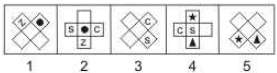Solution:
QUESTION: 50

In the questions, figure (X) is embedded in one of following figures. Find out the correct alternative.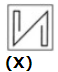Solution: